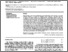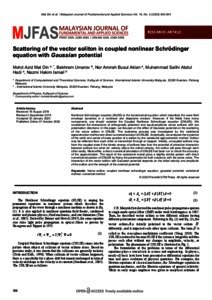# Scattering of the vector soliton in coupled nonlinear Schrödinger equation with Gaussian potential

Mat Din, Mohd Azid and Umarov, Bakhram and Mohd Busul Aklan, Nor Amirah and Abdul Hadi, Muhammad Salihi and Ismail, Nazmi Hakim (2020) Scattering of the vector soliton in coupled nonlinear Schrödinger equation with Gaussian potential. Malaysian Journal of Fundamental and Applied Sciences, 16 (5). pp. 500-504. ISSN 2289-5981 E-ISSN 2289-599XPreview
PDF - Published Version

## Abstract

Nonlinear Schrodinger equation (NLSE) is the fundamental equation which describes the wave field envelope dynamics in a nonlinear and dispersive medium. However, if the fields have many components, one should consider the Coupled Nonlinear Schrodinger equation (CNLSE). We considered the interactions of orthogonally polarized and equal-amplitude vector solitons with two polarization directions. In this paper, we focused on the effect of Gaussian potential on the scattering of the vector soliton in CNLSE. The scattering process was investigated by the variational approximation method and direct numerical solution of CNLSE. Analytically, we analyzed the dynamics of the width and center of mass position of a soliton by the variational approximation method. Soliton may be reflected from each other or transmitted through or trapped. Initially, uncoupled solitons may form the coupled state if the kinetic energy of solitons less than the potential of attractive interaction between solitons but when its’ velocity above the critical velocity, the soliton will pass through each other easily. Meanwhile, a direct numerical simulation of CNLSE had been run to check the accuracy of the approximation. The result of the variational model gives a slightly similar pattern with direct numerical simulation of CNLSE by fixing the parameters for both solutions with the same value. The interaction of the vector soliton with Gaussian potential depends on the initial velocity and amplitude of the soliton and the strength of the external potential.

Item Type: Article (Journal) 3938/86859 Vector soliton, coupled nonlinear schrodinger equation, gaussian potential, variational method, numerical method Q Science > QA Mathematics > QA297 Numerical AnalysisQ Science > QC Physics Kulliyyah of Science > Department of PhysicsKulliyyah of Science > Department of Computational and Theoretical Sciences Dr Bakhram Umarov 28 Dec 2020 15:19 28 Dec 2020 15:19 http://irep.iium.edu.my/id/eprint/86859View Item